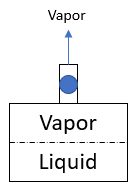## Two-Phase Systems

Consider an insulated storage tank contains an element in a half liquid and half vapor by volume equilibrium state. If a small amount of vapor is released without adding heat, what will happen to the system?

HintHint 2
Specific volume is an intrinsic property of a substance that describes the ratio between a substance's volume (V) to its mass (m).
$$v=\frac{V}{m}$$$The overall mass inside the tank is reduced slightly as vapor is released. Since the tank's vessel maintains its shape, volume is constant. As a result, the specific volume inside the tank must increase to adjust for the lower vapor amount, which can only occur if some liquid evaporates.Remember, specific volume is an intrinsic property of a substance that describes the ratio between a substance's volume (V) to its mass (m). $$v=\frac{V}{m}$$$
Some liquid evaporates.